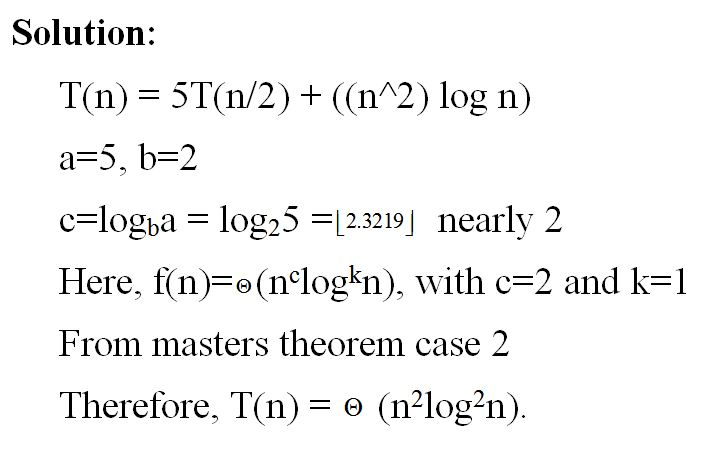# Question & Answer: How to solve the recurrence for T(n) = 5T(n/2) + ((n^2) log n)…..

How to solve the recurrence for T(n) = 5T(n/2) + ((n^2) log n)